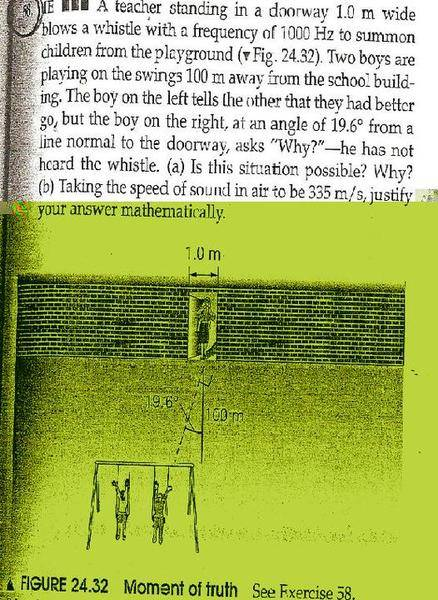# Interesting Wave Problem

bfr

## Homework Statement## Homework Equations

wave velocity = frequency * wave length

## The Attempt at a Solution

Well...I'm not sure. I know how you could find the distance to the person to the right using trigonometric, but I don't know how to actually answer the question (mathematically). Interference might have something to do with it?

Antenna Guy
The width of the doorway (in wavelengths) should be important.

Consider having one whistle, and two echos (one from each side).

Regards,

Bill

Homework Helper

## The Attempt at a Solution

Well...I'm not sure. I know how you could find the distance to the person to the right using trigonometric, but I don't know how to actually answer the question (mathematically). Interference might have something to do with it?

Yes; the sound wave is passing through a wide slit, so this is a single-slit diffracion problem.